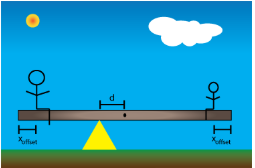# Problem: You have been hired to design a family-friendly see-saw. Your design will feature a uniform board (mass M = 16 kg, length L = 11 m) that can be moved so that the pivot is a distance d from the center of the board. This will allow riders to achieve static equilibrium even if they are of different mass, as most people are. You have decided that each rider will be positioned so that his/her center of mass will be a distance xoffset = 16 cm from the end of the board when seated as shown. You have selected a child of mass m = 29 kg (shown on the right), and an adult of mass n =4 times the mass of the child (shown on the left) to test out your prototype.a) Determine the distance d in m.b) Determine the magnitude of the force exerted on the pivot point by the see-saw in N.

###### FREE Expert Solution

Torque:

$\overline{){\mathbf{\tau }}{\mathbf{=}}{\mathbf{r}}{\mathbf{·}}{\mathbf{Fsin\theta }}}$

The distances and the forces are perpendicular to each other. Thus, θ = 90°.

The weight of the board and the child create clockwise torque about the pivot.

The weight of the adult creates a counterclockwise torque about the pivot.

We'll take counterclockwise as positive.

Στ = 0

94% (289 ratings)###### Problem Details

You have been hired to design a family-friendly see-saw. Your design will feature a uniform board (mass M = 16 kg, length L = 11 m) that can be moved so that the pivot is a distance d from the center of the board. This will allow riders to achieve static equilibrium even if they are of different mass, as most people are. You have decided that each rider will be positioned so that his/her center of mass will be a distance xoffset = 16 cm from the end of the board when seated as shown. You have selected a child of mass m = 29 kg (shown on the right), and an adult of mass n =4 times the mass of the child (shown on the left) to test out your prototype.a) Determine the distance d in m.

b) Determine the magnitude of the force exerted on the pivot point by the see-saw in N.